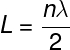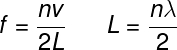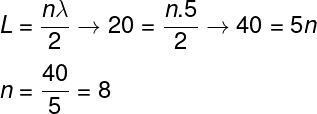Sound and Oscillation

# standing waves

Standing waves are periodic oscillations produced by interference between waves of equal frequency propagating along the same direction but in opposite directions. When an incident wave meets a wave reflected by a fixed end of a string, standing waves, also known as harmonics , are formed . Standing waves are so called because they do not propagate through space . Their oscillations occur exclusively in the perpendicular direction (90º) to the direction of the waves that produced them.

## Characteristics of standing waves

Standing waves, also called harmonics, result from a superposition between different waves . The phenomenon that gives rise to them is resonance . It is for this reason that these waves are used to produce the notes played on string and wind musical instruments, for example.

When a standing wave is formed, some points in space remain at rest all the time. These points are called nodes. Nodes are therefore regions of total destructive interference . In a vibrating string, for example, the formation of a standing wave always gives rise to at least two knots, found at the ends where the string is fixed.

In addition to nodes, standing waves also have antinodes. These points, also called crest and valley, unlike nodes, are points of constructive interference between the two waves and are always in motion.

We call wavelength the distance between three consecutive nodes or the distance between two consecutive antinodes. The wavelength is also the size occupied by a complete oscillation. Furthermore, the measurement of wavelength is limited to the space available for the formation of standing waves, such as the length of the string on which these waves formed.

## Standing waves on strings

Standing waves on strings are formed when an oscillation impinges on the fixed end of a string . When this happens, this wave is reflected with a phase inversion, that is, the wave amplitude has its oscillation and propagation direction reversed. According to the frequency of the wave, the wavelength of the standing waves changes – the higher their frequency, the shorter the wavelength. Furthermore, in order for standing waves to form on strings, it is necessary that the length of the string be an integer multiple (n = 1,2,3,4 ….) of half the wavelength , denoted by the symbol λ.

The formula relating the length of the string (L) to the wavelength (λ) is shown below:n – harmonic order

In the formula above, the integer n is known as the harmonic order . Depending on the size of the string, for a given frequency, there is a finite number of harmonics that can be formed. Among all of them, the lowest frequency harmonic is the harmonic whose n = 1, known as fundamental harmonic .

## Standing waves in sound tubes

Standing waves are also formed when air flows through sound tubes. When this happens, it is possible to produce more intense sounds. There are two types of sound tubes: open tubes and closed tubes. Next, we will learn how to calculate the wavelength and frequency of sound waves in these types of tubes.

### → Wavelength and frequency in open tubes

Open tubes are those that have both ends open. In these tubes, only stationary waves are produced whose antinodes (crest and valley) are formed at the openings of the tube, and the nodes, along its interior.

The formulas used to calculate the wavelength and frequency of the nth standing wave (nth-order harmonic) formed in an open tube of length L are shown below:### → Wavelength and frequency in closed tubes

Closed sound tubes are those that have one of their ends closed. When a standing wave is established in such a tube, nodes are formed along the tube as well as over its capped end. At the open end, a crest and a valley form.

The order of harmonics in closed tubes is determined by an integer and odd multiple (n = 1, 3, 5, 7) of a quarter of the wavelength (λ/4). The formulas used to calculate the wavelength and frequency of a standing wave formed in a closed sound tube of length L are shown below:## Solved exercises on standing waves

Question 1 — Regarding the main characteristics of standing waves, mark the correct alternative.

a) They can only be produced by waves of transverse propagation.

b) They can only be produced by waves of longitudinal propagation.

c) They are characterized by propagating through space with increasing speed.

d) They are formed by the interference between two waves of equal frequencies, which move in opposite directions.

e) They are waves of a mechanical nature, formed exclusively on strings or sound tubes.

Resolution: letter D.

Question 2 — A string of length 20 cm is set to oscillate, being traversed by standing waves of wavelength equal to 5 cm. The harmonic order of this wave is:

a) 2

b) 4

c) 8

d) 3

e) 5

Resolution:

To calculate the harmonic order, we must use the formula that relates the length of the string to the wavelength.